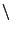Next: 6.2.7 snapshots: get_snap.c and Up: 6.2 The NEMO Macro Previous: 6.2.5 filestruct.h   Contents   Index

## 6.2.6 vectmath.h

The vectmath.h macro package provides a set of macros to handle some elementary operations on two, three or generaldimensional vectors and matrices. The dimensioncan be picked by providing the package with a value for the preprocessor macro NDIM. If this is not supplied, the presence of macros TWODIM and THREEDIM will be checked, in which case NDIM is set to 2 or 3 respectively. The default of NDIM when all of the above are absent, is 3. Of course, the macro NDIM must be provided before vectmath.h is included to have any effect. Resetting the value of NDIM after that, if your compiler would allow it anyhow without an explicit #undef, may produce unpredictable results.

There are also a few of the macro's which can be used as a regular C function, returning a real value, e.g. absv() for the length of a vector.

Operations such as SETV (copying a vector) are properly defined for every dimension, but CROSSVP (a vector cross product) has a different meaning in 2 and 3 dimensions, and is absent in higher dimensions.

It should be noted that the matrices used here are true C matrices, a pointer to an array of pointers (to 1D arrays), unlike FORTRAN arrays, which only occupy a solid 2D block of memory. C arrays take slightly more memory. For an example how to make C arrays and FORTRAN arrays work closely together see e.g. Numerical Recipes in C by Press et al. (MIT Press, 1988).

In the following example a 4 dimensional vector is cleared:

```    #define NDIM 4
#include <vectmath.h>

nemo_main()
{
vector a;           /* same as:   double a  */

CLRV(a);
}
```

some more examples here - taken from some snap codeNext: 6.2.7 snapshots: get_snap.c and Up: 6.2 The NEMO Macro Previous: 6.2.5 filestruct.h   Contents   Index
(c) Peter Teuben